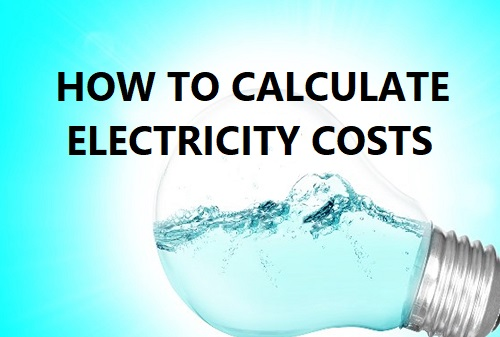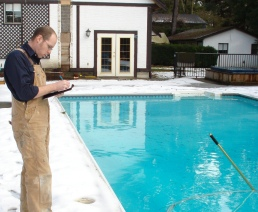• +1 (888) 818 POOL
• swimmingpoolsteve@gmail.com

## How To Calculate Electricity CostsWith news laws coming into effect in 2021 that will force the hand of pool owners to invest into a variable speed pump for their filtration pump more than ever pool owners are interested in knowing just how much electricity savings they can expect. You certainly don't need to look very far to find lofty claims of 80% or more potential savings in electricity but many pool owners want to be able to calculate the savings for themselves. Fortunately it is fairly easy to make these calculations, and once you have done a few you will easily see that variable speed pump technology really does have the potential to drastically reduce the power your pump consumes circulating and filtering your pool water.

The first thing that you will need to know is the price that you pay for your electricity. Electricity is charged based on the kWh (kilowatt-hour) of use however to calculate your real-world costs you will need to read your power bill to learn the different rates for the different times of day. Most areas now have variable electricity rates that are less expensive during off-peak times, typically as low as \$0.07 per kWh as compared to peak rates which can climb as high as \$0.20 to \$0.30 per kWh, or even more if you live in an area with high electricity costs. It is easy enough to use the nationwide average of \$0.131 per kWh to make rough calculations of cost, but in order to be as accurate as possible you would need to know the different rates you pay for the different times of day.

In order to determine your electrical use you need to know the amount of power (in Watts) that your device is consuming. You could know this from using an external Wattage meter as shown in the attached video, or you might have a Wattage meter built in to your appliance as shown with this Pentair SuperFlo VS pool pump. Without the real time Wattage being available you can make a calculation so long as you know both the voltage as well as the current draw. Voltage is the easiest to know as it will be 115 Volts or 230 Volts (approximately) and current can be known by using an amp probe (like a digital multimeter with a claw clamp on one end). Failing this all electrical appliances must be sold with a nameplate that shows the maximum current draw. Without any way to measure the actual current draw you could at least take the maximum current rating from the nameplate. To learn the power consumption (in Watts) simply multiply the voltage (in Volts) by the amperage (in Amps).

Example Electrical Appliance
Voltage = 230 Volts
Amperage = 10 Amps
230 x 10 = 2300 Watts

2300 Watts can also be converted to kilowatts by moving the decimal three places to the left. This would change 2300 Watts into 2.3 kW. That means that this example appliance would consume 2.3kW of power for every hour it operates. Stated another way this appliance uses 2.3 kWh of power. The next step would be to now multiply the kWh power rating by the kWh cost that you pay for electricity. This will give you the cost, in dollars and cents, that you pay for each hour of operation of this appliance.

Off-Peak Rate \$0.07/kWh
2.3 x 0.07 = \$0.161

Nationwide Average Rate \$0.13/kWh
2.3 x 0.13 = \$0.299

Peak Hours Rate \$0.34/kWh
2.3 x 0.34 = \$0.782

With these three examples you can see just how much of a difference peak rate electricity can make versus the much lower off-peak rates. This is one of the advantages of variable speed pool pumps in that you can program it to run at higher speeds where it consumes a great deal of power during the off-peak times of day for electricity rates. Considering that even a few hours of high speed running will use as much or more power than running the pump the remainder of the day on lower speeds you can see why it is important to program your pump properly if you want to experience the maximum electrical savings. For more information you can take a look at this article that talks about variable speed pump schedules.

Important note about Voltage and Current - The best way to calculate electrical costs is to be able to see the current draw in real time, or have a real time Wattage meter to allow you to see either the current draw or power consumption, or both. If you are making calculations based on the current rating on the nameplate then you might not be getting an accurate number. Many heavy load appliances, like and electric motor for a pool pump, will have the highest current draw during the initial startup as it takes a lot of energy to get a stationary motor moving. The current ratings for appliances must show the maximum potential amperage which means that something like a pool pump might run on a continuous current draw lower than the number you see printed on the nameplate. For a real world example of power consumption versus electric motor speeds you can read this article about flow rate and electrical consumption for variable speed pumps.

3 reasons to get a variable speed pool pump

How much electricity does a pool pump use

How does a variable speed pump save you money

Variable speed pump schedules

Variable speed pool pump reviews

Popular variable speed pump modelsIf you want to continue learning about pools and spas from an industry expert follow swimming pool Steve on acebook , and youtube